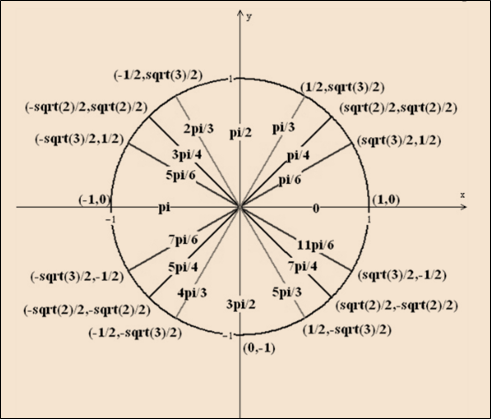# The value of x to satisfy the following equation: 2 cos x − 1 = 0### Single Variable Calculus: Concepts...

4th Edition
James Stewart
Publisher: Cengage Learning
ISBN: 9781337687805### Single Variable Calculus: Concepts...

4th Edition
James Stewart
Publisher: Cengage Learning
ISBN: 9781337687805

#### Solutions

Chapter C, Problem 29E
To determine

## To calculate: The value of x to satisfy the following equation:   2cosx−1=0

Expert Solution

The values of x for the interval [0,2π] to satisfy eq. 2cosx1=0 are π3,5π3 .

### Explanation of Solution

Given information:

2cosx1=0[0,2π]

Calculation:

Know that:

2cosx1=02cosx=1cosx=12

Now, consider the values of x in the given interval [0,2π] that cause cosine value is equal to 12 .

Now, cosx=12 is positive that means it should be in the first and fourth quadrants.

Know that:

The circle has co-ordinates (x,y)=(cosθ,sinθ) with the radius 1 .

Now, the angle corresponding to the x co-ordinates means cosx=12 , a unit circle is drawn as:Fig. Unit circle of cosx=12

From the diagram:

Cosine is equal to 12 when the angle is π3,5π3 .

Therefore, the values of x for the interval [0,2π] to satisfy eq. 2cosx1=0 are π3,5π3 .

### Have a homework question?

Subscribe to bartleby learn! Ask subject matter experts 30 homework questions each month. Plus, you’ll have access to millions of step-by-step textbook answers!